#### contact: vanrij.jacolien "at" gmail "dot" com

Here the data, graphs and analyses scripts (R code) are provided for the paper:
Using context to resolve object pronouns, Jacolien van Rij, Bart Hollebrandse, and Petra Hendriks

Submitted for publication in:
Empirical perspectives on anaphora resolution: Information structural evidence in the race for salience, edited by Anke Holler, Christine Goeb and Katja Suckow.

# Response data

## 1. Data

Participants had to indicate whether the sentence they heard was a correct description of the picture presented on the screen. The responses were yes, no, or missing (labeled as NA).

The response data is available here. Loading data and packages in R:

# Response data:
dat <- readRDS('Data/response_data.rds')

R.version.string
##  "R version 3.2.2 (2015-08-14)"
# For GAMMs:
library(mgcv)
## Loading required package: nlme
## This is mgcv 1.8-7. For overview type 'help("mgcv-package")'.
# For GAMM interpretation and visualization:
library(itsadug)
## Loaded package itsadug 1.0.1 (see 'help("itsadug")' ).
# for generating this R Markdown report the
# info messages are put on:
infoMessages('on')
# load package MASS for calculating inverse later:
library(MASS)
# load package plyr for calculating averages:
library(plyr)
# For printable plot colors:
library(sp)
# Set plot colors:
col1 <- bpy.colors(4)
col2 <- bpy.colors(4)
col3 <- bpy.colors(4)
col4 <- bpy.colors(4)

Note: The GAMM analysis was performed with mgcv version 1.8-3, but this report and the plots were generated with a newer version of mgcv installed.

### Accuracy responses

The leftmost panels of Figure 2 in the paper show dotplots of the average participant means ($$\pm$$ 95%CI) of the responses.

First, the participant means and the 95%CI for the participant means are calculated:

# Calculating average proportion correct per participant and condition:
avg.subj <- with(dat,
aggregate(list(ACC=ACC),
list(Subject=Subject, AgeGroup=AgeGroup, ReferringExpression=ReferringExpression, Context=Context),
mean))

# Calculate averages per group and condition:
avg <- ddply(avg.subj, c("AgeGroup", "ReferringExpression", "Context"),
summarize,
mean=mean(ACC, na.rm=TRUE),
ci = 1.96*se(ACC),
n=length(ACC))
avg$ci.l <- with(avg, mean-ci) avg$ci.u <- with(avg, mean+ci)

This is the code for the dotplots:

# Setup plot window of 8 inch wide and 5 inch heigh
# with quartz(,8,5), dev.new(width=8,height=5) or x11(,8,5)
# depending on the platform.

# Devide plot window in panels:
oldmar <- par()$mar par(mfrow=c(1,2), cex=1.1, mar=c(5,5,4,1)) # Plot info: avg$pch <- with(avg, ifelse(AgeGroup=='Children', 16, 4))
avg$col <- with(avg, ifelse(AgeGroup=='Children', col3, col1)) avg$pos <- with(avg, ifelse(Context=='AP', 3, ifelse(Context=='PA',2,1)))

# Plot 1: Pronouns
emptyPlot(1, c(.5,3.5),
v0=.5,
main="Pronouns", axes=F)
axis(1, at=seq(0,1,by=.5))
mtext(cat <- c('P', 'PA', 'AP'), side=2, at=c(1,2,3), line=.5, cex=1.1, font=1, las=2)
abline(h=c(1,2,3), col=alpha('black', f=.15))
box(col=alpha('black', f=.25))

with(avg[avg$ReferringExpression=='P',], addInterval(pos, ci.l, ci.u, col=col, length=0, xpd=T) ) with(avg[avg$ReferringExpression=='P',],
points(mean, pos, pch=pch, col=col, cex=1.1, lwd=2, xpd=T) )

legend(0, getFigCoords('f'), yjust=0,
pch=c(16,4), col=c(col3, col1), pt.lwd=2,
bty='n', xpd=TRUE)

# Plot 2: Reflexives
emptyPlot(1, c(.5,3.5),
v0=.5,
main="Reflexives", axes=F)
axis(1, at=seq(0,1,by=.5))
mtext(cat <- c('P', 'PA', 'AP'), side=2, at=c(1,2,3), line=.5, cex=1.1, font=1, las=2)
abline(h=c(1,2,3), col=alpha('black', f=.15))
box(col=alpha('black', f=.25))

with(avg[avg$ReferringExpression=='R',], addInterval(pos, ci.l, ci.u, col=col, length=0, xpd=T) ) with(avg[avg$ReferringExpression=='R',],
points(mean, pos, pch=pch, col=col, cex=1.1, lwd=2, xpd=T) )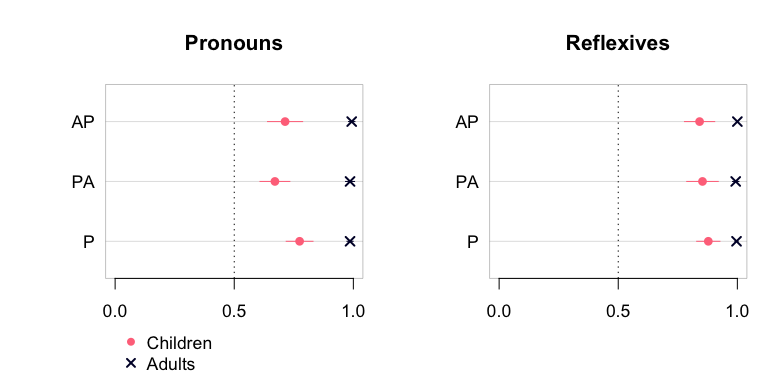## 2. Analysis 1: Single-referent context versus two-referents contexts

Because we tested only four trials per Context condition, we first compared the single-referent context with the two-referent contexts (collapsing AP and PA). In Analysis 2 we will compare the three different contexts and show that the results are comparable.

First, we calculated the SDT measures for the single-referent and the two-referents conditions.

# 1. Re-label responses:
dat$SDT <- with(dat, ifelse(ReferringExpression=='P', ifelse(imageType=='Other' & Response=='yes', "hit", ifelse(imageType=='Self' & Response=='yes', "fa", ifelse(imageType=='Other' & Response=='no', "miss", ifelse(imageType=='Self' & Response=='no', "cr",NA)))), ifelse(imageType=='Self' & Response=='yes', "hit", ifelse(imageType=='Other' & Response=='yes', "fa", ifelse(imageType=='Self' & Response=='no', "miss", ifelse(imageType=='Other' & Response=='no', "cr",NA)))) )) # 2. Count the number of responses in each category: sdt <- with(dat, aggregate(list(counts=SDT), list(Subject=Subject, AgeMonths=AgeMonths, AgeGroup=AgeGroup, ReferringExpression=ReferringExpression, Context=ContextType), function(x){ table(factor(x, levels=c("hit", "fa", "miss", "cr"))) })) # Add total counts: sdt$n <- rowSums(sdt$counts) # 3a. Calculate hit rate and false-alarm rate: sdt$hit.rate <- with(sdt, (counts[,'hit']+.5) / (counts[,'hit']+counts[,'miss']+1))
sdt$fa.rate <- with(sdt, (counts[,'fa']+.5) / (counts[,'fa']+counts[,'cr']+1)) # 3b. Convert hit rate and false alarm rate to z-scores: sdt$z.hit <- qnorm(sdt$hit.rate) sdt$z.fa <- qnorm(sdt$fa.rate) # 4. Calculate d' and C measures in long format: sdt$measure <- 'dp'
sdt$value <- with(sdt, z.hit- z.fa) tmp <- sdt tmp$measure <- 'C'
tmp$value <- with(tmp, -.5*(z.hit+z.fa)) sdt <- rbind(sdt, tmp) sdt <- sdt[sdt$AgeGroup=='Children',]
sdt <- droplevels(sdt)
sdt$Factor <- interaction(sdt$Context, sdt$ReferringExpression, sdt$measure)
levels(sdt$Factor) ##  "1ref.P.C" "2ref.P.C" "1ref.R.C" "2ref.R.C" "1ref.P.dp" "2ref.P.dp" ##  "1ref.R.dp" "2ref.R.dp" sdt$Subject <- as.factor(sdt$Subject) sdt$measure <- as.factor(sdt$measure) sdt$Factor <- as.factor(sdt$Factor) sdt$ReferringExpression <- as.factor(sdt$ReferringExpression) sdt$Context <- as.factor(sdt$Context) Rather than analyzing the measures $$d'$$ and $$C$$ seperately, they are combined in a single analysis following Masson and Kliegl (2013). We only analyzed the data from the child participants, because the adult participants show ceiling performance (low variation in responses). We use three methods to investigate whether and how the predictors Referring Expression, Context (1 ref, 2 ref), and Age (in months) influence the sensitivity $$d'$$ and resoponse bias $$C$$ on the Picture-Verification Task: 1. Using a backward fitting model comparison procedure; 2. Using difference curves and custom contrast coding (cf. Masson and Kliegl 2013); 3. Using plots of the model predictions. [Table of contents] ### Method a: model-comparison procedure # For the SDT data we use gam() rather than bam(), because # the data has less than ten thousand data points. # We start with the full model, including all interactions and # as many random effects as possible. # Note: A random slope for context is not possible, because # then the model has too many parameters for the data. m0 <- gam(value ~ Factor # Factor captures intercept differences + s(AgeMonths, by=Factor) # nonlinear curve for each coondition, fitting the trend over age + s(Subject, bs='re') # random intercept adjustments for participants + s(measure, Subject, bs='re') # random slope adjustements for measure per participant + s(ReferringExpression, Subject, bs='re'), # random slope adjustements for Ref.Expr. per participant data=sdt) The summary below provides the coefficients of the parametric terms (intercept, intercept differences and linear terms) in part “A”, and the smooth terms in part “B”. Note that the parametric-terms summary shows the differences between the conditions and the reference condition (similar to summaries of linear regression models), reflecting the treatment coding that is used by default in R. The smooth summary, in contrast, shows all levels of the categorical predictor Factor, with each line in the smooth summary indicating the trend over Age for that condition. The test statistics of the summary show whether the trend is significantly different from a flat line that includes zero. Hence, the summary does not tell whether the nonlinear effect are different from each other. The summary suggest that any effects of age seem to be present in the $$d'$$ measures, but not in the $$C$$ measure, because the smooths for the $$C$$ measures are not significantly different from a flat line that includes 0. gamtabs(m0, type='HTML')  A. parametric coefficients Estimate Std. Error t-value p-value (Intercept) -0.3462 0.1062 -3.2585 0.0013 Factor2ref.P.C -0.1096 0.0872 -1.2576 0.2099 Factor1ref.R.C 0.2461 0.1053 2.3363 0.0204 Factor2ref.R.C 0.3038 0.1053 2.8849 0.0043 Factor1ref.P.dp 1.6762 0.1160 14.4461 < 0.0001 Factor2ref.P.dp 1.2862 0.1160 11.0849 < 0.0001 Factor1ref.R.dp 2.2233 0.1302 17.0729 < 0.0001 Factor2ref.R.dp 2.0810 0.1302 15.9802 < 0.0001 B. smooth terms edf Ref.df F-value p-value s(AgeMonths):Factor1ref.P.C 1.0000 1.0000 0.0019 0.9657 s(AgeMonths):Factor2ref.P.C 1.0000 1.0000 0.0779 0.7805 s(AgeMonths):Factor1ref.R.C 1.0000 1.0000 0.6874 0.4080 s(AgeMonths):Factor2ref.R.C 1.0000 1.0000 0.0224 0.8811 s(AgeMonths):Factor1ref.P.dp 3.4194 4.0969 2.6085 0.0366 s(AgeMonths):Factor2ref.P.dp 8.1529 8.7397 3.4165 0.0008 s(AgeMonths):Factor1ref.R.dp 1.3110 1.5274 4.8389 0.0101 s(AgeMonths):Factor2ref.R.dp 1.0000 1.0000 16.7082 0.0001 s(Subject) 19.3702 39.0000 18.2238 0.0002 s(measure,Subject) 39.1347 78.0000 5.0903 0.0020 s(ReferringExpression,Subject) 30.7772 78.0000 2.4322 0.0053 Notes: • The summary of parametric coefficients is similar to summaries of linear regression models in R. • The summary of the smooth terms only suggests which smooths are significantly different from 0 (flat line). • The edf are a measure of wigglyness for each smooth function (not the same as the degrees of freedom of the complete model, as presented in the compareML function). An edf of 1 represents a straight line. To investigate whether the interaction between ReferringExpression, Context, and Measure is significant, we used a Chisquare test on the model selection score, with taking into account the degrees of freedom in the model (implemented in the function compareML). Model comparisons showed that the three-way interaction interacting with Age, but not necessary to include as overall (intercept) effect. Example of comparing GAMM models: # Two way interaction factors to replace Factor: sdt$RefMeasure <- interaction(sdt$ReferringExpression, sdt$measure)
sdt$ContextMeasure <- interaction(sdt$Context, sdt$measure) sdt$RefContext <- interaction(sdt$ReferringExpression, sdt$Context)

# Selection of the most important comparisons from the model-comparison procedure:
m1a <- gam(value ~ (ReferringExpression+Context+measure)^3
+ s(AgeMonths, by=RefMeasure)
+ s(Subject, bs='re')
+ s(measure, Subject, bs='re')
+ s(ReferringExpression, Subject, bs='re'),
data=sdt)
# Model m0 is significantly better:
compareML(m0, m1a)

m1b <- gam(value ~ (ReferringExpression+Context+measure)^3
+ s(AgeMonths, by=ContextMeasure)
+ s(Subject, bs='re')
+ s(measure, Subject, bs='re')
+ s(ReferringExpression, Subject, bs='re'),
data=sdt)
# Model m0 is significantly better:
compareML(m0, m1b)

m1c <- gam(value ~ (ReferringExpression+Context+measure)^3
+ s(AgeMonths, by=RefContext)
+ s(Subject, bs='re')
+ s(measure, Subject, bs='re')
+ s(ReferringExpression, Subject, bs='re'),
data=sdt)
# Model m0 is significantly better:
compareML(m0, m1c)

# Delecte the three-way interaction intercept:
m1d <- gam(value ~ (ReferringExpression+Context+measure)^2
+ s(AgeMonths, by=Factor)
+ s(Subject, bs='re')
+ s(measure, Subject, bs='re')
+ s(ReferringExpression, Subject, bs='re'),
data=sdt)
# No difference between m0 and m1d - m1d seems slightly better (lower AIC score):
compareML(m0, m1d)

Summary of the best-fitting model:

gamtabs(m1f, type='HTML')
 A. parametric coefficients Estimate Std. Error t-value p-value (Intercept) -0.3361 0.1039 -3.2341 0.0014 ReferringExpressionR 0.2260 0.0958 2.3592 0.0192 Context2ref -0.1297 0.0753 -1.7218 0.0866 measuredp 1.6561 0.1075 15.4107 < 0.0001 ReferringExpressionR:Context2ref 0.2076 0.0870 2.3862 0.0179 ReferringExpressionR:measuredp 0.3412 0.0870 3.9223 0.0001 Context2ref:measuredp -0.2402 0.0870 -2.7618 0.0062 B. smooth terms edf Ref.df F-value p-value s(AgeMonths):Factor1ref.P.C 1.0000 1.0000 0.0019 0.9657 s(AgeMonths):Factor2ref.P.C 1.0000 1.0000 0.0779 0.7804 s(AgeMonths):Factor1ref.R.C 1.0000 1.0000 0.6881 0.4077 s(AgeMonths):Factor2ref.R.C 1.0000 1.0000 0.0224 0.8810 s(AgeMonths):Factor1ref.P.dp 3.4250 4.1035 2.6147 0.0362 s(AgeMonths):Factor2ref.P.dp 8.1563 8.7414 3.4286 0.0008 s(AgeMonths):Factor1ref.R.dp 1.3112 1.5278 4.8424 0.0100 s(AgeMonths):Factor2ref.R.dp 1.0000 1.0000 16.7245 0.0001 s(Subject) 19.3541 39.0000 18.3557 0.0002 s(measure,Subject) 39.1947 78.0000 5.1383 0.0020 s(ReferringExpression,Subject) 30.8431 78.0000 2.4553 0.0053

#### Conclusions

On the basis of the model fitting procedure we can conclude that there is a significant interaction between measure, Referring Expression and Context, and that these conditions change differently with Age. From the summary, we could conclude that the response bias $$C$$ does not changes with the Age of the particicpants, but some effects of Age are found for the sensitivity $$d'$$.

### Method b: inspection of summary

Because it is difficult to determine on the basis of the summary which factor levels are different from each other, we specified a contrast matrix that allows us to set the comparisons that we are interested in as difference contrasts.

levels(sdt$Factor) ##  "1ref.P.C" "2ref.P.C" "1ref.R.C" "2ref.R.C" "1ref.P.dp" "2ref.P.dp" ##  "1ref.R.dp" "2ref.R.dp" # setup contrast matrix: cmat <- matrix( c(-1/4,-1/4,-1/4,-1/4, 1/4, 1/4, 1/4, 1/4, # dp vs C 0, 0, 0, 0, 1/2, 1/2,-1/2,-1/2, # dp | P vs R 0, 0, 0, 0, 1, -1, 0, 0, # dp | P | 1ref vs 2ref 0, 0, 0, 0, 0, 0, 1, -1, # dp | R | 1ref vs 2ref 1/2, 1/2,-1/2,-1/2, 0, 0, 0, 0, # C | P vs R 1, -1, 0, 0, 0, 0, 0, 0, # C | P | 1ref vs 2ref 0, 0, 1, -1, 0, 0, 0, 0 # C | R | 1ref vs 2ref ), nrow=8, ncol=7, byrow=F) # convert constrasts cmat.i <- fractions(t(ginv(cmat))) # add names: dimnames(cmat.i) <- list(levels(sdt$Factor),
c('_d.C', '_d_P.R', '_d_P_1.2', '_d_R_1.2', '_C_P.R', '_C_P_1.2', '_C_R_1.2'))
# assign contrasts to factor:
sdt$Factor <- C(sdt$Factor, cmat.i, 7)
# inspect contrast:
contrasts(sdt$Factor) ## _d.C _d_P.R _d_P_1.2 _d_R_1.2 _C_P.R _C_P_1.2 _C_R_1.2 ## 1ref.P.C -1/2 0 0 0 1/2 1/2 0 ## 2ref.P.C -1/2 0 0 0 1/2 -1/2 0 ## 1ref.R.C -1/2 0 0 0 -1/2 0 1/2 ## 2ref.R.C -1/2 0 0 0 -1/2 0 -1/2 ## 1ref.P.dp 1/2 1/2 1/2 0 0 0 0 ## 2ref.P.dp 1/2 1/2 -1/2 0 0 0 0 ## 1ref.R.dp 1/2 -1/2 0 1/2 0 0 0 ## 2ref.R.dp 1/2 -1/2 0 -1/2 0 0 0 # As bam()/gam() overrides constrasts for factors, # extract constrasts as separate predictors: mm <- model.matrix( ~ Factor, data=sdt) # and add these to data frame: sdt <- cbind(sdt, mm) After setting up the constrasts, we can run a full model and use the summary to check which contrasts (basically difference curves) are significant: sdt$Subject <- as.factor(sdt$Subject) sdt$measure <- as.factor(sdt$measure) sdt$ReferringExpression <- as.factor(sdt$ReferringExpression) # Now we explicitly add a line for each contrast, # because bam()/gam() will override contrasts for factors. m0diff <- gam(value ~ Factor_d.C + Factor_d_P.R + Factor_d_P_1.2 + Factor_d_R_1.2 + Factor_C_P.R + Factor_C_P_1.2 + Factor_C_R_1.2 + s(AgeMonths, by=Factor_d.C) + s(AgeMonths, by=Factor_d_P.R) + s(AgeMonths, by=Factor_d_P_1.2) + s(AgeMonths, by=Factor_d_R_1.2) + s(AgeMonths, by=Factor_C_P.R) + s(AgeMonths, by=Factor_C_P_1.2) + s(AgeMonths, by=Factor_C_R_1.2) + s(Subject, bs='re') + s(Factor_d.C, Subject, bs='re') + s(Factor_d_P.R, Subject, bs='re') + s(Factor_C_P.R, Subject, bs='re'), data=sdt) # Note that the two full models are not significantly different # in the amount of variation they explain: compareML(m0, m0diff) The summary below provides different information from the summary of model m1e before: the summary still reflect whether the smooths are significantly different from 0, but now the smooths are difference curves. This is the result of the contrast coding. Thus, the summary now tells whether the differences are significant. Based on the summary we can draw the same conclusion: most differences are present in the $$d'$$ measure, less so in the $$C$$ measure. gamtabs(m0diff , type='HTML')  A. parametric coefficients Estimate Std. Error t-value p-value (Intercept) 0.6172 0.0814 7.5832 < 0.0001 Factor_d.C 0.8533 0.0433 19.7273 < 0.0001 Factor_d_P.R -0.4647 0.2778 -1.6732 0.0958 Factor_d_P_1.2 -1.0775 0.9830 -1.0961 0.2743 Factor_d_R_1.2 0.0711 0.0409 1.7407 0.0832 Factor_C_P.R -0.1649 0.0289 -5.7049 < 0.0001 Factor_C_P_1.2 0.0548 0.0409 1.3410 0.1814 Factor_C_R_1.2 -0.0289 0.0409 -0.7068 0.4805 B. smooth terms edf Ref.df F-value p-value s(AgeMonths):Factor_d.C 1.5000 1.5000 107.6536 < 0.0001 s(AgeMonths):Factor_d_P.R 2.6174 2.7427 2.4482 0.1744 s(AgeMonths):Factor_d_P_1.2 8.2382 9.0941 2.2700 0.0121 s(AgeMonths):Factor_d_R_1.2 1.5000 1.5000 3.6717 0.1530 s(AgeMonths):Factor_C_P.R 1.5000 1.5000 9.4684 0.0004 s(AgeMonths):Factor_C_P_1.2 1.5000 1.5000 0.8561 0.4709 s(AgeMonths):Factor_C_R_1.2 1.5000 1.5000 0.9803 0.4759 s(Subject) 37.4781 40.0000 14.7912 < 0.0001 s(Factor_d.C,Subject) 30.2941 39.0000 3.3951 < 0.0001 s(Factor_d_P.R,Subject) 29.3143 39.0000 3.2000 < 0.0001 s(Factor_C_P.R,Subject) 0.0000 41.0000 0.0000 0.5246 [Table of contents] ### Method c: Visualizing the model predictions Finally, we use the predictions of the model to visualize the differences between conditions. Below are the summed effects plotted, which include the intercept differences and smooth effects. The random effects were removed from these plots (with argument rm.ranef=TRUE). Example of the code for plotting the summed effects: par(mfrow=c(2,2), cex=1.1) # Sensitivity of reflexives: plot_smooth(m0, view='AgeMonths', cond=list(Factor="1ref.R.dp"), ylim=c(0,3), h0=NULL, v0=c(5,6)*12, main='Reflexives', ylab="Sensitivity (d')", rug=FALSE) plot_smooth(m0, view='AgeMonths', cond=list(Factor="2ref.R.dp"), col='red', add=TRUE, rug=FALSE) # Same for the other plots, but change levels of Factor...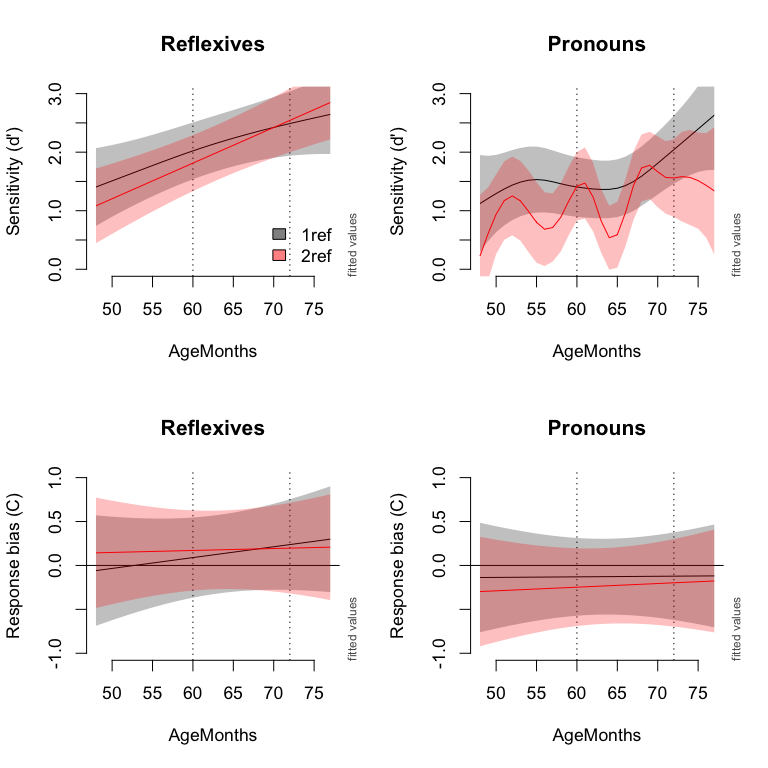These plots show that: • There is no significant change of $$C$$ with age. • $$C$$ is not significantly different from 0 for reflexives and only a small negative negative bias $$C$$ for pronouns; in other words, child participants were highly certain in their answers, not much of a “yes”-bias. • The sensitivity $$d'$$ increases with age on reflexives, with almost adult-like performance for older children. • There are no differences between context conditions, except in the sensitivity $$d'$$ for pronoun interpretation: a lot of variation in the sensitivity $$d'$$ after the two-referents context, but less so after the single-referent context. The differences between the context conditions are visualized below using the plot_diff function. Context only makes a difference, although a small difference, for the sensitivity $$d'$$ in pronoun interpretation (blue line in left picture). Code for plotting the differences between conditions: par(mfrow=c(1,2), cex=1.1) plot_diff(m1f, view='AgeMonths', comp=list(Factor=c('1ref.P.dp','2ref.P.dp')), cond=list(ReferringExpression='P', measure='dp'), lty=1, ylim=c(-.5,1), col='blue', main="d'", ylab="d' value", rm.ranef=TRUE, print.summary=FALSE, xpd=TRUE) plot_diff(m1f, view='AgeMonths', comp=list(Factor=c('1ref.R.dp','2ref.R.dp')), cond=list(ReferringExpression='R', measure='dp'), lty=2, add=TRUE, rm.ranef=TRUE, print.summary=FALSE, xpd=TRUE) # Same code is used for the second plot. # Change levels of Factor and levels of measure.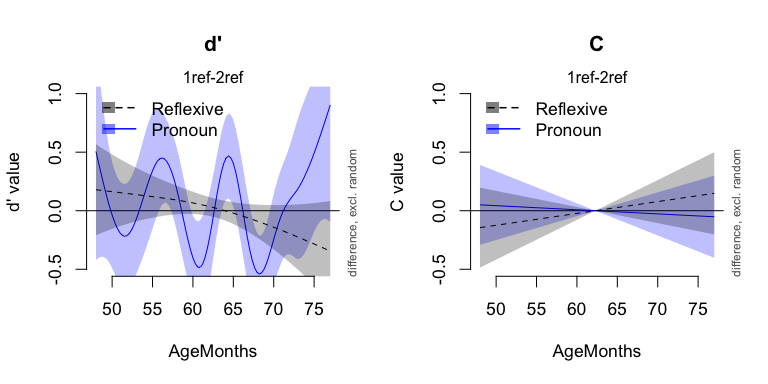Conclusion: Context only seem to affect the children’s sensitivity on pronoun interpretation. [Table of contents] ### Model criticism To evaluate the statistical model, we check the residuals for outliers, distribution, and autocorrelation with the function check_resid. check_resid(m1f, split_by=c("Subject"))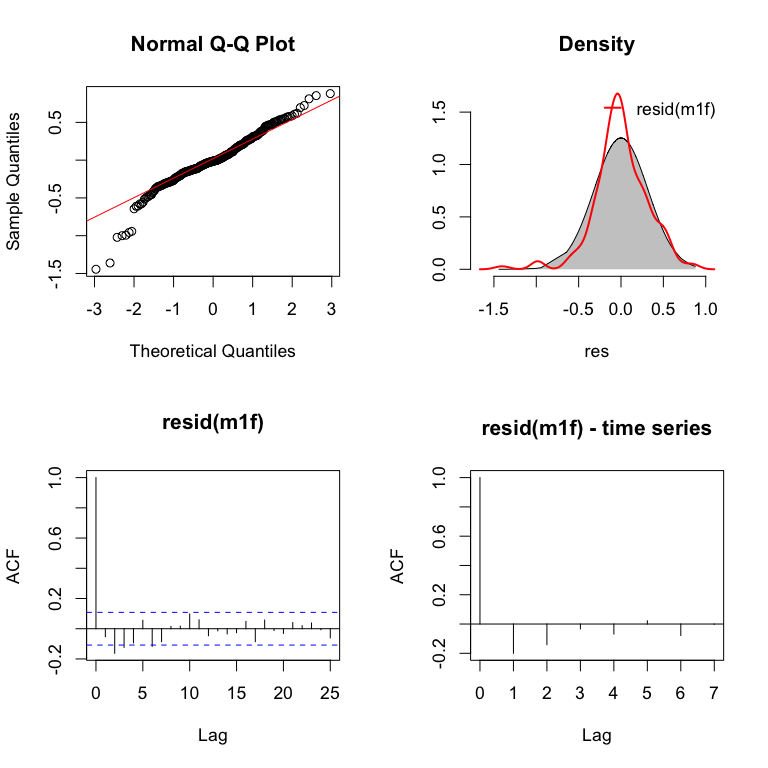The residuals suggest some outliers. We have removed the outliers, and have run te best fitting models again with the data without the outliers. Removing the outliers did not change the results. # Count number of outliers: nrow(sdt[which(resid(m1f) < -.9),]) # Remove outliers: sdt.1 <- sdt[which(resid(m1f) > -.9),] [Table of contents] ### Update: new analysis Note: this additional analysis was performed when preparing the supplementary materials, after the paper was send to the publisher. Therefore this analysis was not reported in the paper. In the previous analyses, the method was set to the default the smoothing parameter selection score ‘GCV’. But actually, the method ‘ML’ is preferred for this data, because it is slightly more conservative as it penalizes wigglyness more than ‘REML’ and ‘GCV’. Although the analyses with method ‘ML’ results in slightly different model predictions and plots, the conclusions do not change. #### * Model comparison procedure: When using method ‘ML’, the model comparison procedure results in a much simpler model: # starting model: m0.ml <- gam(value ~ Factor # Factor captures intercept differences + s(AgeMonths, by=Factor) # nonlinear curve for each coondition, fitting the trend over age + s(Subject, bs='re') # random intercept adjustments for participants + s(measure, Subject, bs='re') # random slope adjustements for measure per participant + s(ReferringExpression, Subject, bs='re'), # random slope adjustements for Ref.Expr. per participant data=sdt, method='ML') # (feel free to do the model comparisons yourself) # preferred model after model comparisons: m1.ml <- gam(value ~ (ReferringExpression+Context+measure)^2 + s(AgeMonths, by=measure) + s(Subject, bs='re') + s(measure, Subject, bs='re') + s(ReferringExpression, Subject, bs='re'), data=sdt, method='ML') compareML(m0.ml, m1.ml) #### * Inspection of the summary: m2.ml <- gam(value ~ Factor_d.C + Factor_d_P.R + Factor_d_P_1.2 + Factor_d_R_1.2 + Factor_C_P.R + Factor_C_P_1.2 + Factor_C_R_1.2 + s(AgeMonths, by=Factor_d.C) + s(AgeMonths, by=Factor_d_P.R) + s(AgeMonths, by=Factor_d_P_1.2) + s(AgeMonths, by=Factor_d_R_1.2) + s(AgeMonths, by=Factor_C_P.R) + s(AgeMonths, by=Factor_C_P_1.2) + s(AgeMonths, by=Factor_C_R_1.2) + s(Subject, bs='re') + s(Factor_d.C, Subject, bs='re') + s(Factor_d_P.R, Subject, bs='re') + s(Factor_C_P.R, Subject, bs='re'), data=sdt, mthod='ML') gamtabs(m2.ml , type='HTML')  A. parametric coefficients Estimate Std. Error t-value p-value (Intercept) 0.6172 0.0814 7.5832 < 0.0001 Factor_d.C 0.8533 0.0433 19.7273 < 0.0001 Factor_d_P.R -0.4647 0.2778 -1.6732 0.0958 Factor_d_P_1.2 -1.0775 0.9830 -1.0961 0.2743 Factor_d_R_1.2 0.0711 0.0409 1.7407 0.0832 Factor_C_P.R -0.1649 0.0289 -5.7049 < 0.0001 Factor_C_P_1.2 0.0548 0.0409 1.3410 0.1814 Factor_C_R_1.2 -0.0289 0.0409 -0.7068 0.4805 B. smooth terms edf Ref.df F-value p-value s(AgeMonths):Factor_d.C 1.5000 1.5000 107.6536 < 0.0001 s(AgeMonths):Factor_d_P.R 2.6174 2.7427 2.4482 0.1744 s(AgeMonths):Factor_d_P_1.2 8.2382 9.0941 2.2700 0.0121 s(AgeMonths):Factor_d_R_1.2 1.5000 1.5000 3.6717 0.1530 s(AgeMonths):Factor_C_P.R 1.5000 1.5000 9.4684 0.0004 s(AgeMonths):Factor_C_P_1.2 1.5000 1.5000 0.8561 0.4709 s(AgeMonths):Factor_C_R_1.2 1.5000 1.5000 0.9803 0.4759 s(Subject) 37.4781 40.0000 14.7912 < 0.0001 s(Factor_d.C,Subject) 30.2941 39.0000 3.3951 < 0.0001 s(Factor_d_P.R,Subject) 29.3143 39.0000 3.2000 < 0.0001 s(Factor_C_P.R,Subject) 0.0000 41.0000 0.0000 0.5246 Note that the same contrasts are significant as in the GCV model. #### * Visualizing the model predictions: However, the predictions of the model are slightly different: The most obvious difference is that the nonlinear pattern in the sensitivity (d’) of the 2 referent pronoun is no longer supported. par(mfrow=c(2,2), cex=1.1) # Sensitivity on reflexives plot_smooth(m1.ml, view='AgeMonths', cond=list(Context='1ref', measure='dp', ReferringExpression='R'), rm.ranef=TRUE, rug=TRUE, lty=2, ylim=c(0,3), h0=NULL, main="d' | Reflexive", ylab="d' value") plot_smooth(m1.ml, view='AgeMonths', cond=list(Context='2ref', measure='dp', ReferringExpression='R'), rm.ranef=TRUE, rug=TRUE, col='red', add=TRUE) # Same for other plots, change levels of Context, measure, and ReferringExpression.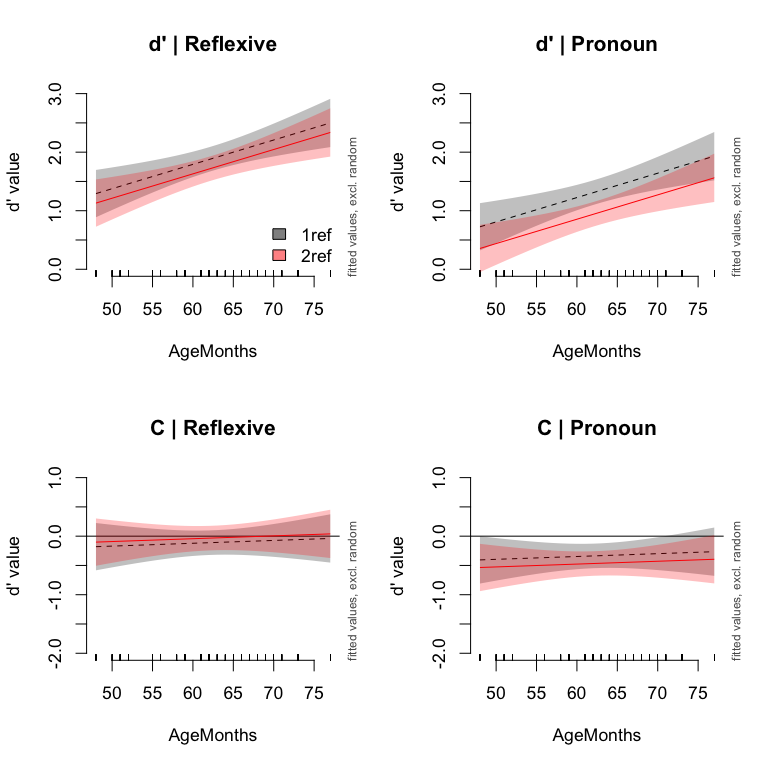Visual inspection of the differences between the two context conditions: par(mfrow=c(1,2), cex=1.1) plot_diff(m1.ml, view='AgeMonths', comp=list(Context=c('1ref', '2ref')), cond=list(ReferringExpression='P', measure="dp"), lty=1, ylim=c(-.5,1), col='blue', main="d'", ylab="d' value", rm.ranef=TRUE, print.summary=FALSE, xpd=TRUE) plot_diff(m1.ml, view='AgeMonths', comp=list(Context=c('1ref', '2ref')), cond=list(ReferringExpression='R', measure='dp'), lty=2, add=TRUE, rm.ranef=TRUE, print.summary=FALSE, xpd=TRUE) # Same for the other plot, different levels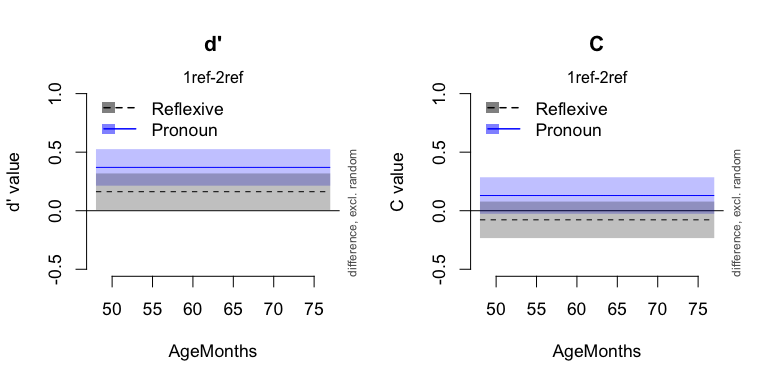Importantly, the new analysis result in similar conclusions: • Only the sensitivity $$d'$$ changes with the Age of the particicpants, but not the response bias $$C$$. • Context seems to affect pronoun processing (significant difference for sensitivity, but not for response bias), but not reflexive processing. [Table of contents] ## 3. Analysis 2: Comparing the three context conditions AP, PA, and P Although we did not test many items for the different context conditions, we compared the three conditions in a similar analysis as in Analysis 1, so that we can compare the results. The granularity of the SDT measures is determined by the number of items per conditions. The single referent context P has 8 items per condition, but the two-referent contexts AP and PA each have only 4 items per condition. To avoid differences in the measures due to the number of items per condition, we randomly divided the items of context P in two groups per subject, P1 and P2. First, we re-calculated the SDT measures for the three context conditions: # 1. Re-label responses in P as P1 and P2 (randomly assign): test <- split(dat, f=list(dat$Subject, dat$ReferringExpression, dat$imageType), drop=TRUE)
dat2 <- do.call('rbind',lapply(test, function(x){
x$newContext <- NA ind <- which(x$Context=="P")

out <- sample(rep(c(0,1),nrow(x[ind,])%/%2))
if(nrow(x[ind,])%%2==1){
out <- c(out, rbinom(1,1,.5))
}
x[ind,]$newContext <- paste("P", out+1, sep='') x[-ind,]$newContext <- x[-ind,]$Context return(x) }) ) row.names(dat2) <- NULL # 2. Count the number of responses in each category: sdt <- with(dat2, aggregate(list(counts=SDT), list(Subject=Subject, AgeMonths=AgeMonths, AgeGroup=AgeGroup, ReferringExpression=ReferringExpression, Context=newContext), function(x){ table(factor(x, levels=c("hit", "fa", "miss", "cr"))) })) # Add total counts: sdt$n <- rowSums(sdt$counts) # Remove all participant-condition combinations with less than 4 items: nrow(sdt[sdt$n < 4,])
##  19
nrow(sdt[sdt$n < 4,])*100/nrow(sdt) ##  3.084416 sdt <- sdt[sdt$n >= 4,]

# 3a. Calculate hit rate and false-alarm rate:
sdt$hit.rate <- with(sdt, (counts[,'hit']+.5) / (counts[,'hit']+counts[,'miss']+1)) sdt$fa.rate <- with(sdt, (counts[,'fa']+.5) / (counts[,'fa']+counts[,'cr']+1))

# 3b. Convert hit rate and false alarm rate to z-scores:
sdt$z.hit <- qnorm(sdt$hit.rate)
sdt$z.fa <- qnorm(sdt$fa.rate)

# 4. Calculate d' and C measures in long format:
sdt$measure <- 'dp' sdt$value <- with(sdt, z.hit- z.fa)

tmp <- sdt
tmp$measure <- 'C' tmp$value <- with(tmp, -.5*(z.hit+z.fa))

sdt <- rbind(sdt, tmp)

sdt$ContextAvg <- gsub('[0-9]+', '', sdt$Context)

sdt$Factor1 <- interaction(sdt$ContextAvg, sdt$ReferringExpression, sdt$measure)
levels(sdt$Factor1) ##  "AP.P.C" "P.P.C" "PA.P.C" "AP.R.C" "P.R.C" "PA.R.C" "AP.P.dp" ##  "P.P.dp" "PA.P.dp" "AP.R.dp" "P.R.dp" "PA.R.dp" sdt$Factor2 <- interaction(sdt$Context, sdt$ReferringExpression, sdt$measure) levels(sdt$Factor2)
##   "AP.P.C"  "P1.P.C"  "P2.P.C"  "PA.P.C"  "AP.R.C"  "P1.R.C"  "P2.R.C"
##   "PA.R.C"  "AP.P.dp" "P1.P.dp" "P2.P.dp" "PA.P.dp" "AP.R.dp" "P1.R.dp"
##  "P2.R.dp" "PA.R.dp"
sdt$Subject <- as.factor(sdt$Subject)
sdt$measure <- as.factor(sdt$measure)
sdt$ReferringExpression <- as.factor(sdt$ReferringExpression)
sdt$Context <- as.factor(sdt$Context)

Figure 2 in the paper presents the averages for the sensitivity and the response bias. The following code was used to calculate the averages:

# Calculating average proportion correct per participant and condition:
avg.subj <- with(sdt[sdt$measure=="dp",], aggregate(list(dp=value), list(Subject=Subject, AgeGroup=AgeGroup, ReferringExpression=ReferringExpression, Context=ContextAvg), mean)) # Calculate averages per group and condition: avg <- ddply(avg.subj, c("AgeGroup", "ReferringExpression", "Context"), summarize, mean=mean(dp, na.rm=TRUE), ci = 1.96*se(dp), n=length(dp)) avg$ci.l <- with(avg, mean-ci)
avg$ci.u <- with(avg, mean+ci) And the code for the first plot: # Setup plot window of 8 inch wide and 5 inch heigh # with quartz(,8,5), dev.new(width=8,height=5) or x11(,8,5) # depending on the platform. # Devide plot window in panels: oldmar <- par()$mar
par(mfrow=c(2,2), cex=1.1, mar=c(5,5,4,1))

# Plot info:
avg$pch <- with(avg, ifelse(AgeGroup=='Children', 16, 4)) avg$col <- with(avg, ifelse(AgeGroup=='Children', col3, col1))
avg$pos <- with(avg, ifelse(Context=='AP', 3, ifelse(Context=='PA',2,1))) # Plot 1: Pronouns emptyPlot(2, c(.5,3.5), v0=0, main="d' | Pronouns", axes=F) axis(1, at=seq(0,2,by=1)) mtext(cat <- c('P', 'PA', 'AP'), side=2, at=c(1,2,3), line=.5, cex=1.1, font=1, las=2) abline(h=c(1,2,3), col=alpha('black', f=.15)) box(col=alpha('black', f=.25)) with(avg[avg$ReferringExpression=='P',],
addInterval(pos, ci.l, ci.u, col=col, length=0, xpd=T) )
with(avg[avg$ReferringExpression=='P',], points(mean, pos, pch=pch, col=col, cex=1.1, lwd=2, xpd=T) ) # The other panels are being plotted in the same way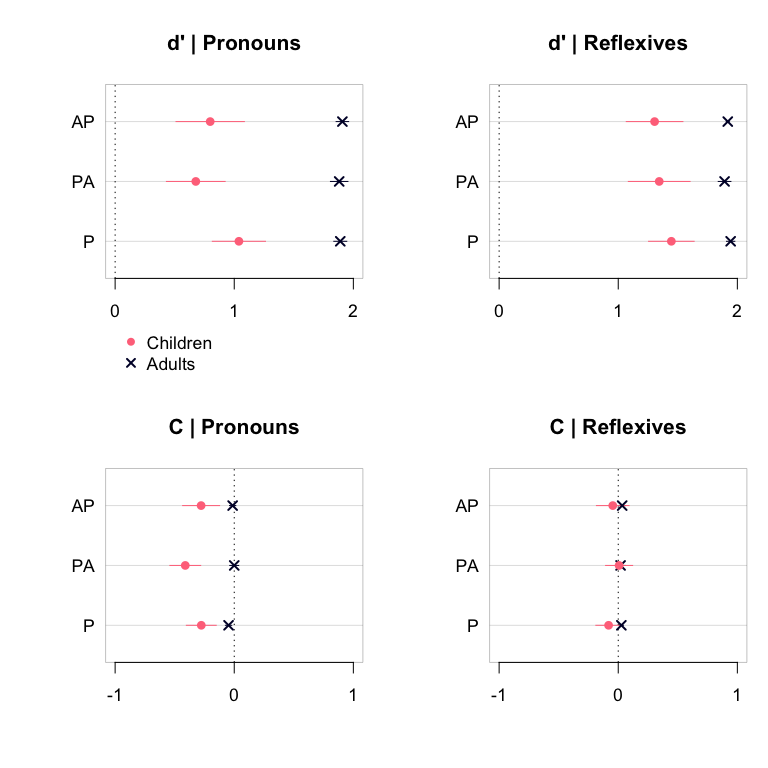[Table of contents] Again, we used the same three analysis methods to test for the effects of Context: 1. Using a backward fitting model comparison procedure; 2. Using difference curves and custom contrast coding (cf. Masson and Kliegl 2013); 3. Using plots of the model predictions. ### Method a: model-comparison procedure We only modeled chilren’s data, as adults show ceiling performance. sdt <- sdt[sdt$AgeGroup=='Children',]
sdt <- droplevels(sdt)

sdt$Subject <- as.factor(sdt$Subject)
sdt$measure <- as.factor(sdt$measure)
sdt$ReferringExpression <- as.factor(sdt$ReferringExpression)
sdt$ContextAvg <- as.factor(sdt$ContextAvg)

# relevel factors, to end up with the right contrasts:
sdt$ReferringExpression <- relevel(sdt$ReferringExpression, ref='R')
sdt$measure <- relevel(sdt$measure, ref='dp')
sdt$ContextAvg <- relevel(sdt$ContextAvg, ref='P')

Setting up a GAMM model:

# We start with the full model, including all interactions and
# as many random effects as possible.

# Note: A random slope for context is not possible, because
# then the model has too many parameters for the data.

m0 <- gam(value ~ Factor1 # <- intercept differences
# nonlinear curve for each coondition, fitting the trend over age:
+ s(AgeMonths, by=Factor1)
# random intercept adjustments for participants:
+ s(Subject, bs='re')
# random slope adjustements for measure per participant:
+ s(measure, Subject, bs='re')
# random slope adjustements for Ref.Expr. per participant:
+ s(ReferringExpression, Subject, bs='re'),
data=sdt)

This model shows a similar pattern as the models before: most intercept differences and Age effects in the sensitivity $$d'$$, but not in the measure $$C$$.

gamtabs(m0, type='HTML')
 A. parametric coefficients Estimate Std. Error t-value p-value (Intercept) -0.2781 0.0964 -2.8854 0.0041 Factor1P.P.C 0.0115 0.0854 0.1344 0.8932 Factor1PA.P.C -0.1328 0.0979 -1.3558 0.1758 Factor1AP.R.C 0.2323 0.1088 2.1355 0.0332 Factor1P.R.C 0.1913 0.0973 1.9653 0.0499 Factor1PA.R.C 0.2854 0.1088 2.6231 0.0090 Factor1AP.P.dp 1.0772 0.1122 9.6049 < 0.0001 Factor1P.P.dp 1.3140 0.1014 12.9539 < 0.0001 Factor1PA.P.dp 0.9555 0.1121 8.5195 < 0.0001 Factor1AP.R.dp 1.5855 0.1218 13.0220 < 0.0001 Factor1P.R.dp 1.7332 0.1116 15.5251 < 0.0001 Factor1PA.R.dp 1.6248 0.1218 13.3444 < 0.0001 B. smooth terms edf Ref.df F-value p-value s(AgeMonths):Factor1AP.P.C 1.0000 1.0000 0.0072 0.9322 s(AgeMonths):Factor1P.P.C 1.0000 1.0000 0.0178 0.8939 s(AgeMonths):Factor1PA.P.C 1.0000 1.0000 0.2070 0.6493 s(AgeMonths):Factor1AP.R.C 1.0000 1.0000 0.0465 0.8294 s(AgeMonths):Factor1P.R.C 1.0000 1.0000 1.1029 0.2941 s(AgeMonths):Factor1PA.R.C 1.0000 1.0000 0.0050 0.9435 s(AgeMonths):Factor1AP.P.dp 8.0700 8.7288 3.4652 0.0003 s(AgeMonths):Factor1P.P.dp 2.5659 3.0688 2.0004 0.1204 s(AgeMonths):Factor1PA.P.dp 6.8342 7.8769 1.2546 0.2184 s(AgeMonths):Factor1AP.R.dp 2.3450 2.9004 6.8609 0.0002 s(AgeMonths):Factor1P.R.dp 1.9614 2.3630 3.8374 0.0136 s(AgeMonths):Factor1PA.R.dp 1.6558 2.0094 7.7347 0.0005 s(Subject) 20.2994 38.0000 18.2940 < 0.0001 s(measure,Subject) 34.5352 76.0000 3.6184 0.0022 s(ReferringExpression,Subject) 30.2931 76.0000 2.5042 0.0018

Model comparsion procedure:

# Starting with intercept differences:
m1a <- gam(value ~ (ReferringExpression+ContextAvg+measure)^3
+ s(Subject, bs='re')
+ s(measure, Subject, bs='re')
+ s(ReferringExpression, Subject, bs='re'),
data=sdt)
m1b <- gam(value ~ (ReferringExpression+ContextAvg+measure)^2
+ s(Subject, bs='re')
+ s(measure, Subject, bs='re')
+ s(ReferringExpression, Subject, bs='re'),
data=sdt)
# 3-way interaction not significant:
compareML(m1a, m1b)
m1c <- gam(value ~ ReferringExpression+ContextAvg+measure
+ s(Subject, bs='re')
+ s(measure, Subject, bs='re')
+ s(ReferringExpression, Subject, bs='re'),
data=sdt)
# 2-way interaction(s) significant:
compareML(m1b, m1c)

# Further with age effects:
m1c <- gam(value ~ (ReferringExpression+ContextAvg+measure)^2
+ s(AgeMonths)
+ s(Subject, bs='re')
+ s(measure, Subject, bs='re')
+ s(ReferringExpression, Subject, bs='re'),
data=sdt)
# No general effect of Age:
compareML(m1b, m1c)

sdt$RefMeasure <- with(sdt, interaction(ReferringExpression, measure)) m1c <- gam(value ~ (ReferringExpression+ContextAvg+measure)^2 + s(AgeMonths, by=RefMeasure) + s(Subject, bs='re') + s(measure, Subject, bs='re') + s(ReferringExpression, Subject, bs='re'), data=sdt) # Significant: compareML(m1b, m1c) sdt$ContextMeasure <- with(sdt, interaction(ContextAvg, measure))
m1d <- gam(value ~ (ReferringExpression+ContextAvg+measure)^2
+ s(AgeMonths, by=ContextMeasure)
+ s(Subject, bs='re')
+ s(measure, Subject, bs='re')
+ s(ReferringExpression, Subject, bs='re'),
data=sdt)
# Marginally significant:
compareML(m1b, m1d)

m1e <- gam(value ~ (ReferringExpression+ContextAvg+measure)^2
+ s(AgeMonths, by=Factor1)
+ s(Subject, bs='re')
+ s(measure, Subject, bs='re')
+ s(ReferringExpression, Subject, bs='re'),
data=sdt)
# Significantly better:
compareML(m1c, m1e)

# Check whether 1ref / 2ref is better than AP,PA, P:
sdt$ContextNew <- as.factor(with(sdt, ifelse(as.character(ContextAvg)=="P",'1ref', '2ref'))) sdt$Factor3 <- with(sdt, interaction(ReferringExpression, ContextNew, measure))

m1g <- gam(value ~ (ReferringExpression+ContextNew+measure)^2
+ s(AgeMonths, by=Factor3)
+ s(Subject, bs='re')
+ s(measure, Subject, bs='re')
+ s(ReferringExpression, Subject, bs='re'),
data=sdt)
# m1e significantly better:
compareML(m1e, m1g)

Thus, splitting the data in AP,PA,and P is preferred over 1ref vs 2ref Contexts:

m1e <- gam(value ~ (ReferringExpression+ContextAvg+measure)^2
+ s(AgeMonths, by=Factor1)
+ s(Subject, bs='re')
+ s(measure, Subject, bs='re')
+ s(ReferringExpression, Subject, bs='re'),
data=sdt)
gamtabs(m1e, type='HTML')
 A. parametric coefficients Estimate Std. Error t-value p-value (Intercept) 1.4562 0.0814 17.8888 < 0.0001 ReferringExpressionP -0.4190 0.0781 -5.3646 < 0.0001 ContextAvgAP -0.1573 0.0730 -2.1555 0.0316 ContextAvgPA -0.1107 0.0730 -1.5167 0.1300 measureC -1.5437 0.0813 -18.9935 < 0.0001 ReferringExpressionP:ContextAvgAP -0.0691 0.0844 -0.8190 0.4132 ReferringExpressionP:ContextAvgPA -0.2455 0.0844 -2.9091 0.0038 ReferringExpressionP:measureC 0.2416 0.0690 3.4988 0.0005 ContextAvgAP:measureC 0.2061 0.0843 2.4446 0.0148 ContextAvgPA:measureC 0.2078 0.0843 2.4649 0.0140 B. smooth terms edf Ref.df F-value p-value s(AgeMonths):Factor1AP.P.C 1.0000 1.0000 0.0072 0.9324 s(AgeMonths):Factor1P.P.C 1.0000 1.0000 0.0077 0.9301 s(AgeMonths):Factor1PA.P.C 1.0000 1.0000 0.2087 0.6479 s(AgeMonths):Factor1AP.R.C 1.0000 1.0000 0.0472 0.8281 s(AgeMonths):Factor1P.R.C 1.0000 1.0000 0.8890 0.3462 s(AgeMonths):Factor1PA.R.C 1.0000 1.0000 0.0051 0.9430 s(AgeMonths):Factor1AP.P.dp 8.0107 8.6963 3.3023 0.0006 s(AgeMonths):Factor1P.P.dp 3.0854 3.6878 2.1510 0.1127 s(AgeMonths):Factor1PA.P.dp 6.9027 7.9300 1.3419 0.1841 s(AgeMonths):Factor1AP.R.dp 2.3216 2.8670 6.8762 0.0003 s(AgeMonths):Factor1P.R.dp 1.9631 2.3568 4.3461 0.0082 s(AgeMonths):Factor1PA.R.dp 1.7553 2.1392 7.5290 0.0005 s(Subject) 19.4855 38.0000 18.0304 < 0.0001 s(measure,Subject) 34.8061 76.0000 3.7907 0.0018 s(ReferringExpression,Subject) 31.8983 76.0000 2.9316 0.0019

### Method b: inspection of summary

levels(sdt$Factor1) ##  "AP.P.C" "P.P.C" "PA.P.C" "AP.R.C" "P.R.C" "PA.R.C" "AP.P.dp" ##  "P.P.dp" "PA.P.dp" "AP.R.dp" "P.R.dp" "PA.R.dp" # setup contrast matrix: cmat <- matrix( c(-1/6,-1/6,-1/6,-1/6,-1/6,-1/6, 1/6, 1/6, 1/6, 1/6, 1/6, 1/6, # dp vs C 0, 0, 0, 0, 0, 0, 1/3, 1/3, 1/3,-1/3,-1/3,-1/3, # dp | P vs R 0, 0, 0, 0, 0, 0, 1, 0, -1, 0, 0, 0, # dp | P | AP vs PA 0, 0, 0, 0, 0, 0, 0, 1, -1, 0, 0, 0, # dp | P | P vs PA 0, 0, 0, 0, 0, 0, 0, 0, 0, 1, 0, -1, # dp | R | AP vs PA 0, 0, 0, 0, 0, 0, 0, 0, 0, 0, 1, -1, # dp | R | P vs PA 1/3, 1/3, 1/3,-1/3,-1/3,-1/3, 0, 0, 0, 0, 0, 0, # C | P vs R 1, 0, -1, 0, 0, 0, 0, 0, 0, 0, 0, 0, # C | P | AP vs PA 0, 1, -1, 0, 0, 0, 0, 0, 0, 0, 0, 0, # C | P | P vs PA 0, 0, 0, 1, 0, -1, 0, 0, 0, 0, 0, 0, # C | R | AP vs PA 0, 0, 0, 0, 1, -1, 0, 0, 0, 0, 0, 0 # C | R | P vs PA ), nrow=12, ncol=11, byrow=F) # convert constrasts cmat.i <- fractions(t(ginv(cmat))) # add names: dimnames(cmat.i) <- list(levels(sdt$Factor1),
c('_d.C', '_d_P.R', '_d_P_AP.PA', '_d_P_P.PA', '_d_R_AP.PA','_d_R_P.PA',
'_C_P.R', '_C_P_AP.PA', '_C_P_P.PA', '_C_R_AP.PA','_C_R_P.PA'))
# assign contrasts to factor:
sdt$Factor1 <- C(sdt$Factor1, cmat.i, 11)
# inspect contrast:
contrasts(sdt$Factor1) ## _d.C _d_P.R _d_P_AP.PA _d_P_P.PA _d_R_AP.PA _d_R_P.PA _C_P.R ## AP.P.C -1/2 0 0 0 0 0 1/2 ## P.P.C -1/2 0 0 0 0 0 1/2 ## PA.P.C -1/2 0 0 0 0 0 1/2 ## AP.R.C -1/2 0 0 0 0 0 -1/2 ## P.R.C -1/2 0 0 0 0 0 -1/2 ## PA.R.C -1/2 0 0 0 0 0 -1/2 ## AP.P.dp 1/2 1/2 2/3 -1/3 0 0 0 ## P.P.dp 1/2 1/2 -1/3 2/3 0 0 0 ## PA.P.dp 1/2 1/2 -1/3 -1/3 0 0 0 ## AP.R.dp 1/2 -1/2 0 0 2/3 -1/3 0 ## P.R.dp 1/2 -1/2 0 0 -1/3 2/3 0 ## PA.R.dp 1/2 -1/2 0 0 -1/3 -1/3 0 ## _C_P_AP.PA _C_P_P.PA _C_R_AP.PA _C_R_P.PA ## AP.P.C 2/3 -1/3 0 0 ## P.P.C -1/3 2/3 0 0 ## PA.P.C -1/3 -1/3 0 0 ## AP.R.C 0 0 2/3 -1/3 ## P.R.C 0 0 -1/3 2/3 ## PA.R.C 0 0 -1/3 -1/3 ## AP.P.dp 0 0 0 0 ## P.P.dp 0 0 0 0 ## PA.P.dp 0 0 0 0 ## AP.R.dp 0 0 0 0 ## P.R.dp 0 0 0 0 ## PA.R.dp 0 0 0 0 # As bam()/gam() overrides constrasts for factors, # extract constrasts as separate predictors: mm <- model.matrix( ~ Factor1, data=sdt) # and add these to data frame: sdt <- cbind(sdt, mm) sdt$Subject <- as.factor(sdt$Subject) sdt$ReferringExpression <- as.factor(sdt\$ReferringExpression)

m0diff <- gam(value ~ Factor1_d.C
+ Factor1_d_P.R + Factor1_d_P_AP.PA + Factor1_d_P_P.PA
+ Factor1_d_R_AP.PA + Factor1_d_R_P.PA
+ Factor1_C_P.R + Factor1_C_P_AP.PA + Factor1_C_P_P.PA
+ Factor1_C_R_AP.PA + Factor1_C_R_P.PA
+ s(AgeMonths, by=Factor1_d.C)
+ s(AgeMonths, by=Factor1_d_P.R)
+ s(AgeMonths, by=Factor1_d_P_AP.PA)
+ s(AgeMonths, by=Factor1_d_P_P.PA)
+ s(AgeMonths, by=Factor1_d_R_AP.PA)
+ s(AgeMonths, by=Factor1_d_R_P.PA)
+ s(AgeMonths, by=Factor1_C_P.R)
+ s(AgeMonths, by=Factor1_C_P_AP.PA)
+ s(AgeMonths, by=Factor1_C_P_P.PA)
+ s(AgeMonths, by=Factor1_C_R_AP.PA)
+ s(AgeMonths, by=Factor1_C_R_P.PA)
+ s(Subject, bs='re')
+ s(Factor1_d.C, Subject, bs='re')
+ s(Factor1_d_P.R, Subject, bs='re')
+ s(Factor1_C_P.R, Subject, bs='re')
+ s(ReferringExpression, Subject, bs='re'),
data=sdt)
gamtabs(m0diff, type='HTML')
 A. parametric coefficients Estimate Std. Error t-value p-value (Intercept) 0.4606 0.0653 7.0529 < 0.0001 Factor1_d.C 0.6428 0.0332 19.3406 < 0.0001 Factor1_d_P.R -0.3485 0.1467 -2.3758 0.0179 Factor1_d_P_AP.PA 0.2063 0.9203 0.2241 0.8228 Factor1_d_P_P.PA 0.1798 0.0417 4.3139 < 0.0001 Factor1_d_R_AP.PA 0.2948 0.3091 0.9539 0.3406 Factor1_d_R_P.PA 0.2357 0.1731 1.3620 0.1738 Factor1_C_P.R -0.1390 0.0260 -5.3504 < 0.0001 Factor1_C_P_AP.PA 0.0664 0.0477 1.3903 0.1650 Factor1_C_P_P.PA 0.0696 0.0416 1.6707 0.0954 Factor1_C_R_AP.PA -0.0265 0.0477 -0.5554 0.5788 Factor1_C_R_P.PA -0.0477 0.0414 -1.1497 0.2508 B. smooth terms edf Ref.df F-value p-value s(AgeMonths):Factor1_d.C 1.5000 1.5000 102.1914 < 0.0001 s(AgeMonths):Factor1_d_P.R 2.1373 2.2517 2.8530 0.1985 s(AgeMonths):Factor1_d_P_AP.PA 8.1702 9.0452 3.4267 0.0005 s(AgeMonths):Factor1_d_P_P.PA 1.5000 1.5000 5.0314 0.0080 s(AgeMonths):Factor1_d_R_AP.PA 3.4812 4.2294 2.4951 0.0430 s(AgeMonths):Factor1_d_R_P.PA 2.6108 3.1539 2.0387 0.0992 s(AgeMonths):Factor1_C_P.R 1.5000 1.5000 8.7628 0.0007 s(AgeMonths):Factor1_C_P_AP.PA 1.5000 1.5000 0.9857 0.4407 s(AgeMonths):Factor1_C_P_P.PA 1.5000 1.5000 1.2124 0.3446 s(AgeMonths):Factor1_C_R_AP.PA 1.5000 1.5000 0.1555 0.8329 s(AgeMonths):Factor1_C_R_P.PA 1.5000 1.5000 1.7768 0.3240 s(Subject) 35.9722 39.0000 14.5168 < 0.0001 s(Factor1_d.C,Subject) 27.7589 38.0000 2.7323 < 0.0001 s(Factor1_d_P.R,Subject) 26.6021 38.0000 2.7171 < 0.0001 s(Factor1_C_P.R,Subject) 0.0000 40.0000 0.0000 0.8893 s(ReferringExpression,Subject) 3.2912 77.0000 0.0674 0.1834

The model shows generally the same picture as the models before. Interestingly, there seems to be a highly significant difference betweenthe AP and PA conditions on the sensitivity $$d'$$.

mdiff <- gam(value ~ Factor1_d.C
+ Factor1_d_P.R + Factor1_d_P_P.PA
+ Factor1_C_P.R
+ s(AgeMonths, by=Factor1_d.C)
+ s(AgeMonths, by=Factor1_d_P_AP.PA)
+ s(Subject, bs='re')
+ s(Factor1_d.C, Subject, bs='re')
+ s(Factor1_d_P.R, Subject, bs='re')
+ s(Factor1_C_P.R, Subject, bs='re'),
data=sdt)

### Method c: visualizing the model predictions

The estimates of the best-fitting model, model m1e, are visualized for reflexives and pronouns for the sensitivity and the response bias. The 95% CI are canceled, because they are largely overlapping.

Below the code is given for one of the four plots. The other plots are generated in the same way.

par(mfrow=c(2,2), cex=1.1)

context <- c("AP", "PA", "P")
ltys <- c(1,5,3)
cols <- c(col1, col2, col3)

# Reflexives, dp
emptyPlot(c(50,77), 2,
v0=c(4,5,6)*12,
main="Reflexives", ylab="sensitivity (d')",
xlab='')

mtext(paste(c(4,5,6), 'yrs'), side=1, line=3.5, at=(c(4,5,6)+.5)*12, font=3, xpd=TRUE)

re <- 'R'
m  <- 'dp'

for(i in 1:3){
plot_smooth(m1e, view='AgeMonths',
cond=list(Factor1=sprintf("%s.%s.%s",context[i],re, m),
ReferringExpression=re, measure=m,
ContextAvg=context[i]),
col=cols[i], lwd=i, lty=ltys[i], se=-1,
}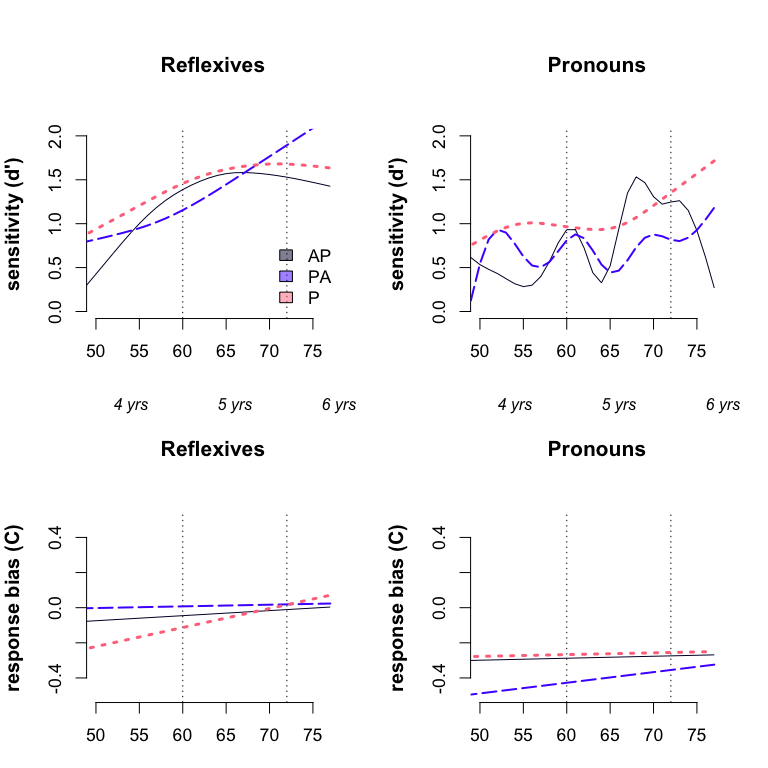# Reflexives, dp
emptyPlot(c(50,